• Home
• Services
• Industries
• Technologies
• Projects
• News
• Blog
• Career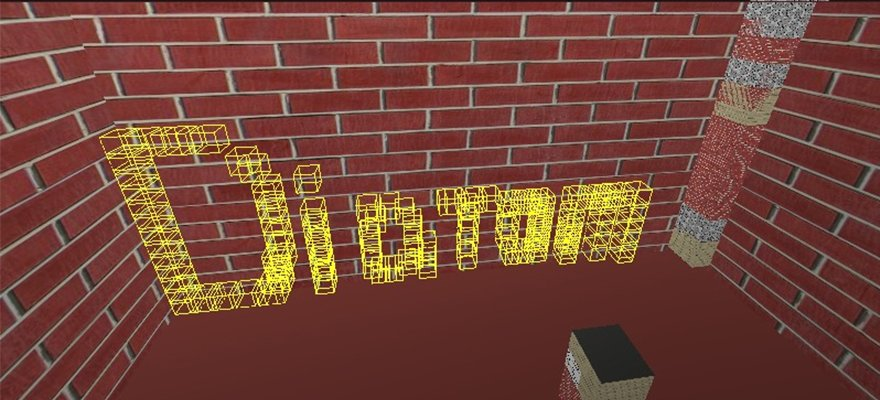BLOG

# Different sides of Ruby development: OpenGL

OpenGL is common everywhere and learning it once, you will be able to build projects with it on any language. The same is with Ruby.

When I’m trying to take a break from web development, I like to build something new and uncommon in Ruby programming. Ruby is typically known for web services, APIs and other web-related development. But with some time, you can build many other types of software.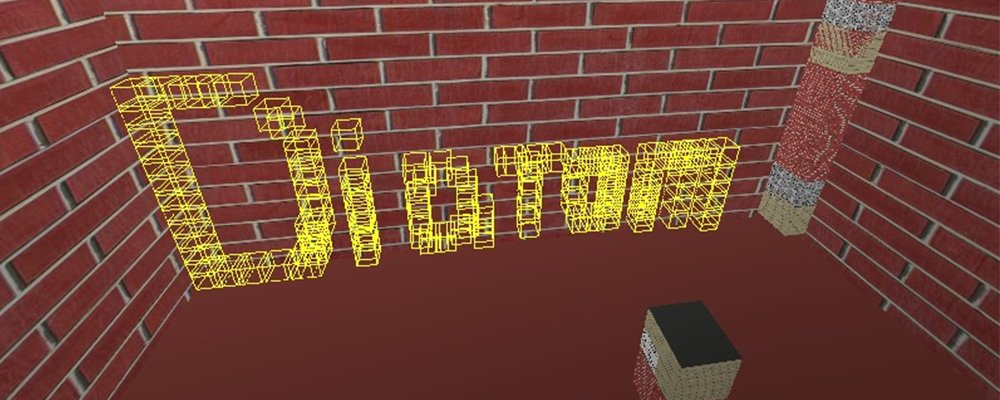After creating, I started researching Ruby applications that use 3D graphics. I found a nice library, OpenGL for Ruby, and created a few samples with it. At first, it can be difficult to understand everything, but let’s see what can we do!

To start working with OpenGL for Ruby, we only need two gems:

`Gemfile.rb:`

```source "https://rubygems.org"
gem "gosu"
gem "opengl"

```

I chose gosu because it provides good functionality to work with windows, players and partial rendering of window objects.

## Let’s create something simple!

Sometimes it’s hard to imagine how everything should look in 3D, so for the first step, I chose to draw an axis instead of a simple cube.

This is a good way to see how our coordinates lie on the three-dimensional grid. Also, we will set the colour of the lines to define where the x, y and z coordinates are.

I suggest that you separate objects into different classes that will stay under the `objects/` directory. So `media/axis.rb` will contain:

```class Axis

def initialize(x_width, y_width, z_width)
@x_width = x_width
@y_width = y_width
@z_width = z_width
end

def self.draw(x_width, y_width, z_width)
object = new(x_width, y_width, z_width)
object.draw
end

def draw
glBegin(GL_LINES)
glColor3d(1, 0, 0)
glVertex3d(0, 0, 0)
glVertex3d(x_width, 0, 0)
glColor3d(0, 1, 0)
glVertex3d(0, 0, 0)
glVertex3d(0, y_width, 0)
glColor3d(0, 0, 1)
glVertex3d(0, 0, 0)
glVertex3d(0, 0, z_width)
glEnd
end
end

```

All the OpenGL magic happens in the draw action. `glBegin` and `glEnd` works like a ruby block where in argument you need to send the type of object that you want to draw in the argument. You can find all the types in the official documentation.

Inside this block, you set the lines and their colour. This sets the colour for all the lines that you will draw after the method `glColor3d`. There are lots of different implementations of the `glColor` method that differ only by the type of argument.`glColor3d` takes arguments in `GLdouble`, `glColor3f`, `GLfloat`, etc. `glColor3d` takes three arguments – red, green and blue values. `glColor4*` can also take an alpha value.

To draw a line, you need to set two points, and OpenGL will draw a line between them. To do this, you need to call `glVertex`. You can pass two to four arguments of different types, depending on the method you are trying to call. In our case, we are sending the x, y and z coordinates of our future points. You can set as many lines in your `glBegin…glEnd` block as you wish. In my example, I’m drawing lines from the beginning of the axis `(x = 0, y = 0, z = 0)` to `(x = x_width, y = y_width, z = z_width)` in pixels.

```require 'opengl'
require 'glu'
require 'gosu'
Dir["objects/*"].each {|file| require_relative file }
include Gl, Glu

class Window &lt; Gosu::Window

def initialize
super 800, 600
self.caption = "Diatom's OpenGL Tutorial"
end

def update
end

def draw
gl do
glClear(GL_COLOR_BUFFER_BIT | GL_DEPTH_BUFFER_BIT)
Axis.draw(100, 100, 100)
end
end

def button_down(id)
exit if id == Gosu::KbEscape
end
end

Window.new.show

```

`glClear` clears buffers to preset values. After that we call `Axis.draw` to draw our axis.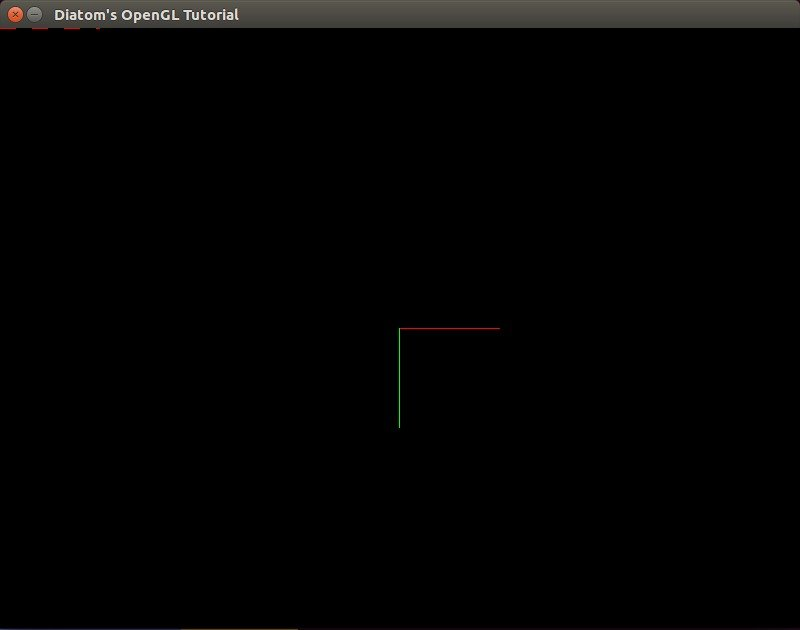As you can see, we can’t find an `y` axis because there is a frame border in its place. So if you want, you can set start of the line from (x = 1) instead of (x = 0).Here we see a problem: what if we want to draw set different objects, but not from the top left corner? What if we want to move to the centre of the axes?

## Moving the centre of the axes

To move to the centre of the axes, we can use the `glTranslate` method. It multiplies the current matrix by a translation matrix and produces a translation by x, y, z. All the objects after the `glTranslate` method will be drawn depending on those coordinates. To work with both translated and normal coordinates, we need to use the `glPushMatrix` and `glPopMatrix` methods, which will save the previous matrix and restore it. `glTranslate` produces different methods – `glTranslated(x, y, z), glTranslatef(x, y, z)` etc. – where x, y and z are the coordinates of a translation vector.

Let’s update our code using this method.

``` def draw
gl do
glClear(GL_COLOR_BUFFER_BIT | GL_DEPTH_BUFFER_BIT)
glPushMatrix
glTranslated(width/2, height/2, 0)
Axis.draw(100, 100, 100)
glPopMatrix
end
end

```As we can now see, our axis moved to the centre of our screen. To see the difference and how `glPushMatrix` and `glPopMatrix` work, let’s add the previous axis too.

``` def draw
gl do
glClear(GL_COLOR_BUFFER_BIT | GL_DEPTH_BUFFER_BIT)

glPushMatrix
glTranslated(width/2, height/2, 0)
Axis.draw(100, 100, 100)
glPopMatrix

glEnable(GL_LINE_STIPPLE)
glLineStipple(2,0x00FF)

Axis.draw(100, 100, 100)
glDisable(GL_LINE_STIPPLE)
end
end

```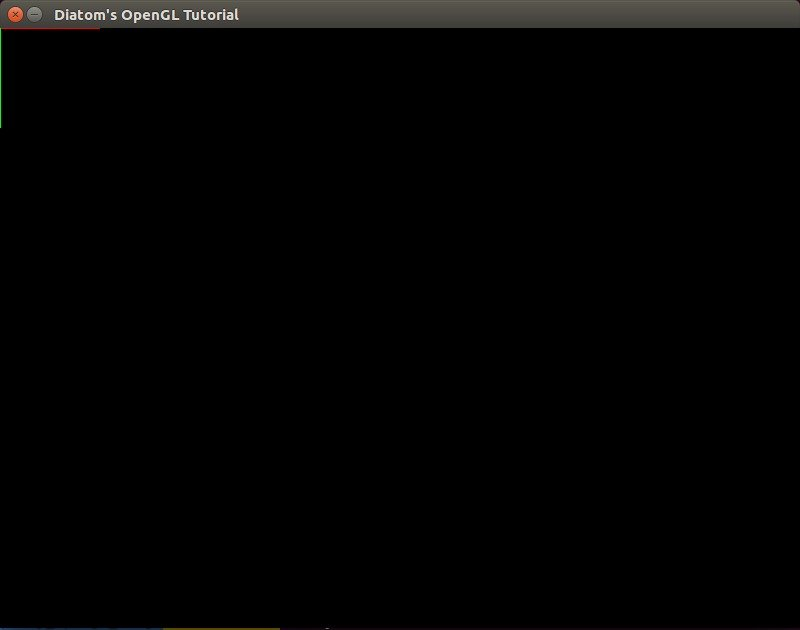As you can see, the previous axis was drawn on the (0, 0, 0) coordinates with the stippled lines without any change to `axis.rb` or its coordinates.

It seems like we forgot about the z coordinate that we can’t see on our flat screen. To take a look at it, we need to use a viewpoint.

## Getting into the real 3D world

At first, I suggest that you build another object on our scene – a cube. It will help us to see the difference between the angles of our viewpoint.

To draw it, we can use `GL_QUADS`. Each of the four points defines a Quad, so to make a cube we need six Quads = 24 points.

`objects/cube.rb:`

```require 'pry'
class Cube
attr_reader :x_width, :y_width, :z_width, :mode, :mode_face

def initialize(x_width, y_width, z_width, mode_face, mode)
@x_width = x_width
@y_width = y_width
@z_width = z_width
@mode = mode
@mode_face = mode_face
end

def self.draw(x_width, y_width, z_width, mode_face = GL_FRONT_AND_BACK, mode = GL_FILL)
object = new(x_width, y_width, z_width, mode_face, mode)
object.draw
end

def draw
glPolygonMode(mode_face, mode);
#yx
glColor3d(1, 0, 0)
glVertex3d(0, 0, 0)
glVertex3d(x_width, 0, 0)
glVertex3d(x_width, y_width, 0)
glVertex3d(0, y_width, 0)
#yz
glColor3d(0, 1, 0)
glVertex3d(0, y_width, 0)
glVertex3d(0, 0, 0)
glVertex3d(0, 0, z_width)
glVertex3d(0, y_width, z_width)
#zx
glColor3d(0, 0, 1)
glVertex3d(0, 0, z_width)
glVertex3d(0, 0, 0)
glVertex3d(x_width, 0, 0)
glVertex3d(x_width, 0, z_width)
#z -&gt; yx
glColor3d(1, 0, 0)
glVertex3d(0, 0, z_width)
glVertex3d(x_width, 0, z_width)
glVertex3d(x_width, y_width, z_width)
glVertex3d(0, y_width, z_width)
#x -&gt; yz
glColor3d(0, 1, 0)
glVertex3d(x_width, y_width, 0)
glVertex3d(x_width, 0, 0)
glVertex3d(x_width, 0, z_width)
glVertex3d(x_width, y_width, z_width)
#y -&gt; zx
glColor3d(0, 0, 1)
glVertex3d(0, y_width, z_width)
glVertex3d(0, y_width, 0)
glVertex3d(x_width, y_width, 0)
glVertex3d(x_width, y_width, z_width)
glEnd
end
end

```

I’ve added two new variables – arguments for `glPolygonMode` that set a rasterization mode. The arguments are face (front, back) and mode (`GL_POINT, GL_LINE, GL_FILL`).

Let’s also update our axes and move code that defines their line types inside.

`objects/axis.rb`:

```class Axis

def initialize(x_width, y_width, z_width, stipple = false)
@x_width = x_width
@y_width = y_width
@z_width = z_width
@stipple = stipple
end

def self.draw(x_width, y_width, z_width, stipple = false)
object = new(x_width, y_width, z_width, stipple)
object.draw
end

def draw
if stipple
glEnable(GL_LINE_STIPPLE)
glLineStipple(2,0x00FF)
end
glBegin(GL_LINES)
...
glEnd
glDisable(GL_LINE_STIPPLE) if stipple
end
end

```

Let’s update our code to use our new cubes.

```def draw
gl do
glClear(GL_COLOR_BUFFER_BIT | GL_DEPTH_BUFFER_BIT)

glPushMatrix
glTranslated(width/2, height/2, 0)
Axis.draw(100, 100, 100)
Cube.draw(50, 50, 50, GL_FRONT_AND_BACK, GL_LINE)
glPopMatrix

Cube.draw(50, 50, 50, GL_FRONT_AND_BACK, GL_LINE)

Axis.draw(100, 100, 100, true)
end
end

```Looks clear, doesn’t it?

To add perspective, we need to use the `gluPerspective` method, which is used to set up a perspective projection. It’s done once to set up how the scene will be rendered. If `gluPerspective` is used, the perspective correction will happen while rendering. The arguments are the angle of view, aspect ratio, z-near and z-far.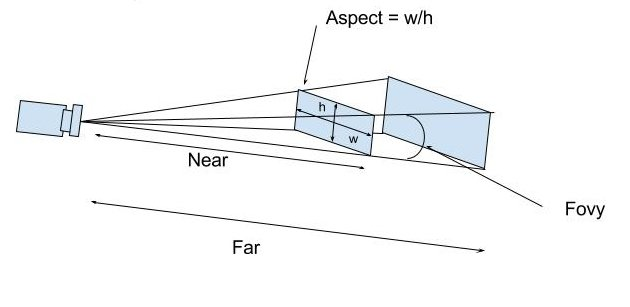So, let’s add perspective to our application.

```def draw
gl do
glClear(GL_COLOR_BUFFER_BIT | GL_DEPTH_BUFFER_BIT)

glMatrixMode(GL_PROJECTION)
gluPerspective(130, width.to_f/height, 0, 500)
glMatrixMode(GL_MODELVIEW)

glPushMatrix
glTranslated(width/2, height/2, 0)
Axis.draw(100, 100, 100)
Cube.draw(50, 50, 50, GL_FRONT_AND_BACK, GL_LINE)
glPopMatrix

Cube.draw(50, 50, 50, GL_FRONT_AND_BACK, GL_LINE)

Axis.draw(100, 100, 100, true)
end
end

```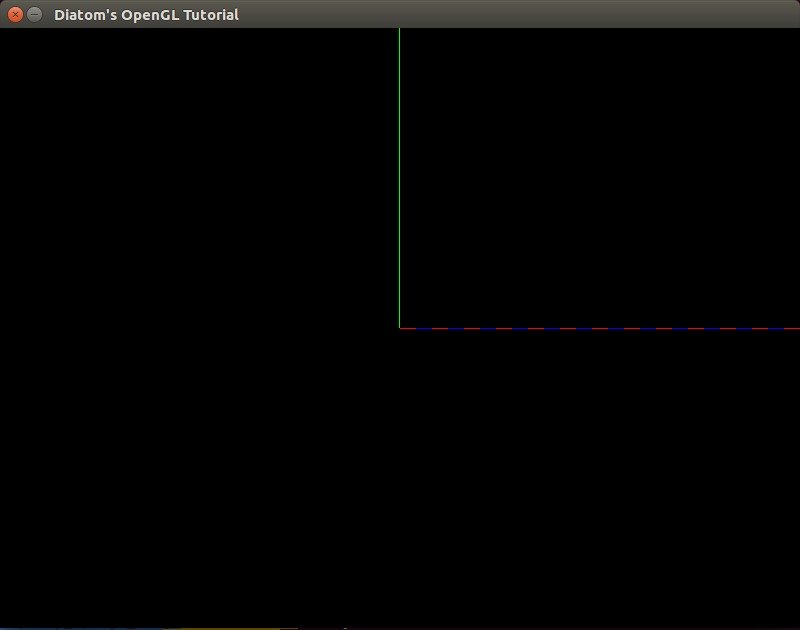We can see that our field of view has been updated, and we can see only part of our (0, 0, 0) cube and axis. To see what `gluPerspective` really changes, let’s add a viewpoint.

To turn the player, OpenGL developers usually build a matrix of the view, load it and use later to turn the entire scene around the player. To simplify our code, we will create a ‘camera’ object that will help us look at the whole scene. To do this, we will use `gluLookAt`.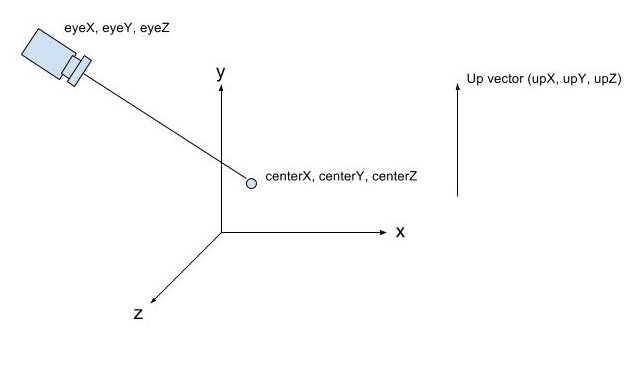If we don’t set `gluLookAt`, OpenGL will automatically set it to (x = 0, y = 0, z = 0). Looking at our screen, that will be equal to `gluLookAt(0, 0, 1, 0, 0, 0, 0, 1, 0)`. So, let’s set our `gluLookAt` properly, so we can see our scene.

```  glMatrixMode(GL_MODELVIEW)
<b>gluLookAt(400, 400, 400, 0, 0, 0, 0, 1, 0)</b>

```

`gluLookAt` sets our camera to point (x = 400, y = 400, z = 400) and looks at point (x = 0, y = 0, z = 0), three other coordinates of `up` vector that we will not touch for now.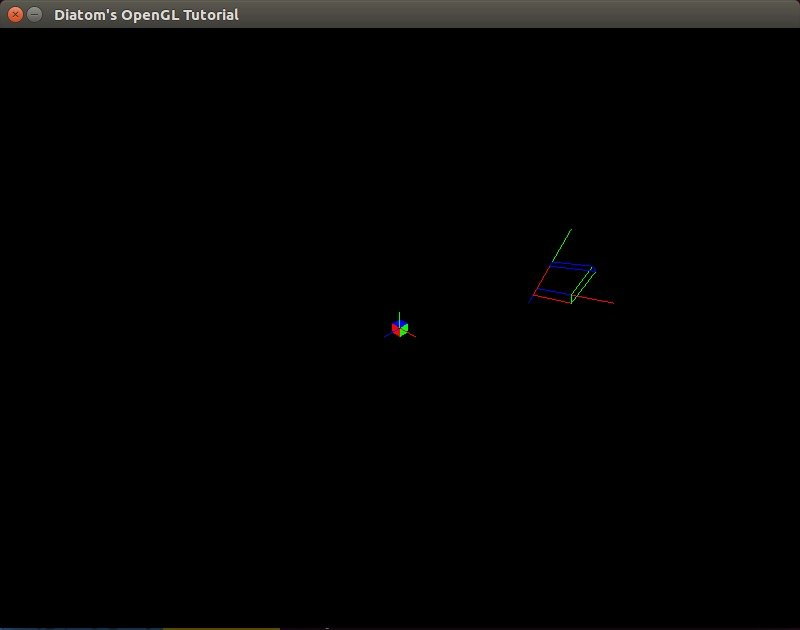Let’s play with gluPerspective a bit now.

`gluPerspective(130, width.to_f/height, 0.001, 225)`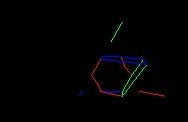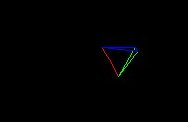What if we want to move the camera and see the other sides of our scene? Here gosu helps with its built-in I\O mechanisms.

## Moving the camera

As you noticed, we can change all of the arguments of the `gluLookAt` method. To move our camera, we need to bind those changes to the keyboard.

I will not explain the difficult math behind the matrixes and real turning of cameras as it was made in games\applications through formulas of radiuses and angles. I want to move our camera only through axes. I know that it’s not the best solution, but it’s the easiest for understanding.

Let’s move our `gluLookAt` method inside the new camera object that will be in objects/camera.rb

```class Camera

def initialize(window)
@window = window
@camera_position = Vector3.new(400, 400, 400)
@look_at_object_position = Vector3.new(0, 0, 0)
@speed = 10
end

def capture
font = Gosu::Font.new(window, Gosu::default_font_name, 20)
button_down
font.draw("Camera position: #{camera_position.x}:#{camera_position.y}:#{camera_position.z}", 10, 10, 3.0, 1.0, 1.0, 0xffffffff)
font.draw("Look at position: #{look_at_object_position.x}:#{look_at_object_position.y}:#{look_at_object_position.z}", 10, 25, 3.0, 1.0, 1.0, 0xffffffff)
font.draw("Your speed: #{speed}", 10, 40, 3.0, 1.0, 1.0, 0xffffffff)
gluLookAt(camera_position.x, camera_position.y, camera_position.z, look_at_object_position.x, look_at_object_position.y, look_at_object_position.z, 0, 1, 0)
end

def button_down
@camera_position.x += speed if Gosu::button_down?(Gosu::KbRight) &amp;&amp; !Gosu::button_down?(Gosu::KB_LEFT_SHIFT)
@camera_position.x -= speed if Gosu::button_down?(Gosu::KbLeft) &amp;&amp; !Gosu::button_down?(Gosu::KB_LEFT_SHIFT)
@camera_position.y += speed if Gosu::button_down?(Gosu::KbUp) &amp;&amp; !Gosu::button_down?(Gosu::KB_LEFT_SHIFT)
@camera_position.y -= speed if Gosu::button_down?(Gosu::KbDown) &amp;&amp; !Gosu::button_down?(Gosu::KB_LEFT_SHIFT)
@camera_position.z += speed if Gosu::button_down?(Gosu::KB_NUMPAD_8) &amp;&amp; !Gosu::button_down?(Gosu::KB_LEFT_SHIFT)
@camera_position.z -= speed if Gosu::button_down?(Gosu::KB_NUMPAD_2) &amp;&amp; !Gosu::button_down?(Gosu::KB_LEFT_SHIFT)

@look_at_object_position.x += speed if Gosu::button_down?(Gosu::KbRight) &amp;&amp; Gosu::button_down?(Gosu::KB_LEFT_SHIFT)
@look_at_object_position.x -= speed if Gosu::button_down?(Gosu::KbLeft) &amp;&amp; Gosu::button_down?(Gosu::KB_LEFT_SHIFT)
@look_at_object_position.y += speed if Gosu::button_down?(Gosu::KbUp) &amp;&amp; Gosu::button_down?(Gosu::KB_LEFT_SHIFT)
@look_at_object_position.y -= speed if Gosu::button_down?(Gosu::KbDown) &amp;&amp; Gosu::button_down?(Gosu::KB_LEFT_SHIFT)
@look_at_object_position.z += speed if Gosu::button_down?(Gosu::KB_NUMPAD_8) &amp;&amp; Gosu::button_down?(Gosu::KB_LEFT_SHIFT)
@look_at_object_position.z -= speed if Gosu::button_down?(Gosu::KB_NUMPAD_2) &amp;&amp; Gosu::button_down?(Gosu::KB_LEFT_SHIFT)
end
end

```

To understand how everything is working, I bind each axis to a key. Here is the schema to move the camera:

```UP, DOWN - by Y axis
LEFT, RIGHT - by X axis
NUM8, NUM2 - by Z axis

```

To move the target point, you need to hold shift, clicking on the same buttons.

I can display the current coordinates of points and the current camera speed using gosu’s text output methods. To do this, we need to pass a ‘window’ object into our class and work with it. For camera position, I wrote a Vector3 class that contains x, y and z coordinates:
objects/vector3.rb:

``` Vector3 = Struct.new(:x, :y, :z)

```

After we build our camera class, let’s use it inside run.rb.

```  def draw
gl do
glClear(GL_COLOR_BUFFER_BIT | GL_DEPTH_BUFFER_BIT)

glMatrixMode(GL_PROJECTION)
gluPerspective(90, width.to_f/height, 0, 500)
glMatrixMode(GL_MODELVIEW)

@camera.capture

glPushMatrix
glTranslated(width/2, height/2, 0)
Axis.draw(100, 100, 100)
Cube.draw(50, 50, 50, GL_FRONT_AND_BACK, GL_LINE)
glPopMatrix

Cube.draw(50, 50, 50, GL_FRONT_AND_BACK, GL_FILL)

Axis.draw(100, 100, 100, true)

end
end

```

‘Fly’ a bit on our scene to see how `gluLookAt` works.

I suggest rewriting the keyboard settings a bit to make them more useful. Also, I want to be able to increase our camera’s speed. For this I want to rewrite the camera object:
objects/camera.rb

```def button_down
check_speed
case
when Gosu::button_down?(Gosu::KbD)
@camera_position.x += speed
@look_at_object_position.x += speed
when Gosu::button_down?(Gosu::KbA)
@camera_position.x -= speed
@look_at_object_position.x -= speed
when Gosu::button_down?(Gosu::KbW)
@camera_position.z -= speed
@look_at_object_position.z -= speed
when Gosu::button_down?(Gosu::KbS)
@camera_position.z += speed
@look_at_object_position.z += speed
when Gosu::button_down?(Gosu::KbUp)
@look_at_object_position.y += speed
when Gosu::button_down?(Gosu::KbDown)
@look_at_object_position.y -= speed
when Gosu::button_down?(Gosu::KbRight)
@look_at_object_position.x += speed
when Gosu::button_down?(Gosu::KbLeft)
@look_at_object_position.x -= speed
end
end

def check_speed
@speed = 10
@speed = 20 if Gosu::button_down?(Gosu::KB_LEFT_SHIFT)
end

```

Let’s use the W and S keys to move the camera on the z axis, A and D to move the camera on the x axis, UP and DOWN to move the target of view on the y axis and LEFT and RIGHT to move the target of view on the x axis. Holding shift will increase our speed.

## Let’s play!

Let’s add a cube object filled with lines to our pointer. To do this, we need to update our cube code to receive the current coordinates and build a cube depending on them.

objects/cube.rb

```require 'pry'
class Cube
attr_reader :position, :x_width, :y_width, :z_width, :mode, :mode_face

def initialize(x_width, y_width, z_width, mode_face, mode, x = 0, y = 0, z = 0)
@x_width = x_width
@y_width = y_width
@z_width = z_width
@mode = mode
@mode_face = mode_face
@position = Vector3.new(x, y, z)
end

def self.draw(x_width, y_width, z_width, mode_face = GL_FRONT_AND_BACK, mode = GL_FILL, x = 0, y = 0, z = 0)
object = new(x_width, y_width, z_width, mode_face, mode, x, y, z)
object.draw
end

def draw
glPolygonMode(mode_face, mode);
#yx
glColor3d(1, 0, 0)
glVertex3d(position.x, position.y, position.z)
glVertex3d(x_width + position.x, position.y, position.z)
glVertex3d(x_width + position.x, y_width + position.y, position.z)
glVertex3d(position.x, y_width + position.y, position.z)
#yz
glColor3d(0, 1, 0)
glVertex3d(position.x, y_width + position.y, position.z)
glVertex3d(position.x, position.y, position.z)
glVertex3d(position.x, position.y, z_width + position.z)
glVertex3d(position.x, y_width + position.y, z_width + position.z)
#zx
glColor3d(0, 0, 1)
glVertex3d(position.x, position.y, z_width + position.z)
glVertex3d(position.x, position.y, position.z)
glVertex3d(x_width + position.x, position.y, position.z)
glVertex3d(x_width + position.x, position.y, z_width + position.z)
#z -&gt; yx
glColor3d(1, 0, 0)
glVertex3d(position.x, position.y, z_width + position.z)
glVertex3d(x_width + position.x, position.y, z_width + position.z)
glVertex3d(x_width + position.x, y_width + position.y, z_width + position.z)
glVertex3d(position.x, y_width + position.y, z_width + position.z)
#x -&gt; yz
glColor3d(0, 1, 0)
glVertex3d(x_width + position.x, y_width + position.y, position.z)
glVertex3d(x_width + position.x, position.y, position.z)
glVertex3d(x_width + position.x, position.y, z_width + position.z)
glVertex3d(x_width + position.x, y_width + position.y, z_width + position.z)
#y -&gt; zx
glColor3d(0, 0, 1)
glVertex3d(position.x, y_width + position.y, z_width + position.z)
glVertex3d(position.x, y_width + position.y, position.z)
glVertex3d(x_width + position.x, y_width + position.y, position.z)
glVertex3d(x_width + position.x, y_width + position.y, z_width + position.z)

glColor3d(1, 1, 1) #return back primary color
glEnd
end
end

```

Now we can update our main window’s method draw with this code

``` glPushMatrix
glTranslated(width/2, height/2, 0)
Axis.draw(100, 100, 100)
Cube.draw(50, 50, 50, GL_FRONT_AND_BACK, GL_FILL)
Cube.draw(50, 50, 50, GL_FRONT_AND_BACK, GL_LINE, @camera.look_at_object_position.x, @camera.look_at_object_position.y, @camera.look_at_object_position.z)
glPopMatrix

```

At the end, you should see this view: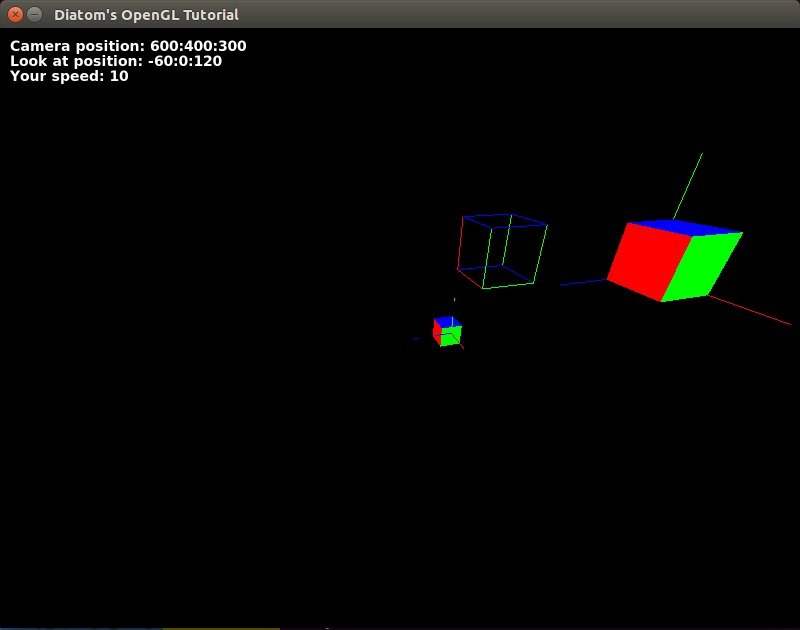## Cube creator

What if we want to place some new cubes? Easy!

Let’s change our run.rb code to

```require 'opengl'
require 'glu'
require 'gosu'

Dir["objects/*"].each {|file| require_relative file }

include Gl, Glu

class Window &lt; Gosu::Window

<b>attr_accessor :scene_objects, :translation</b>

def initialize
super 800, 600
self.caption = "Diatom's OpenGL Tutorial"
@camera = Camera.new(self)
<b>@scene_objects = []
@translation = Vector3.new(width/2, height/2,0)</b>
end

def update
end

def draw
gl do
glClear(GL_COLOR_BUFFER_BIT | GL_DEPTH_BUFFER_BIT)

glMatrixMode(GL_PROJECTION)
gluPerspective(90, width.to_f/height, 0, 500)
glMatrixMode(GL_MODELVIEW)
@camera.capture

<b>@scene_objects &lt;&lt; Axis.new(100, 100, 100)
@scene_objects &lt;&lt; Cube.new(50, 50, 50, GL_FRONT_AND_BACK, GL_FILL)</b>

glPushMatrix
<b>glTranslated(translation.x, translation.y, translation.z)
@scene_objects.each do |object|
object.draw
end</b>
glPopMatrix
Cube.draw(50, 50, 50, GL_FRONT_AND_BACK, GL_LINE, @camera.look_at_object_position.x, @camera.look_at_object_position.y, @camera.look_at_object_position.z)

Cube.draw(50, 50, 50, GL_FRONT_AND_BACK, GL_FILL)

Axis.draw(100, 100, 100, true)
end
end

def button_down(id)
exit if id == Gosu::KbEscape
end
<b>
@scene_objects &lt;&lt; Cube.new(
50, 50, 50,
GL_FRONT_AND_BACK, GL_FILL,
@camera.look_at_object_position.x - translation.x, @camera.look_at_object_position.y - translation.y, @camera.look_at_object_position.z - translation.z
)
end
</b>
end

Window.new.show

```

I have highlighted the changes. As you will notice, I moved the translation vector to the variable of Vector3 class, moved all objects that will be translated and drawn on the scene to the array @scene_objects and then placed them on the scene with a loop.

To add a new cube, I can just create a new cube object and place it in the array. Coordinates should depend on the translation vector to place everything on valid places.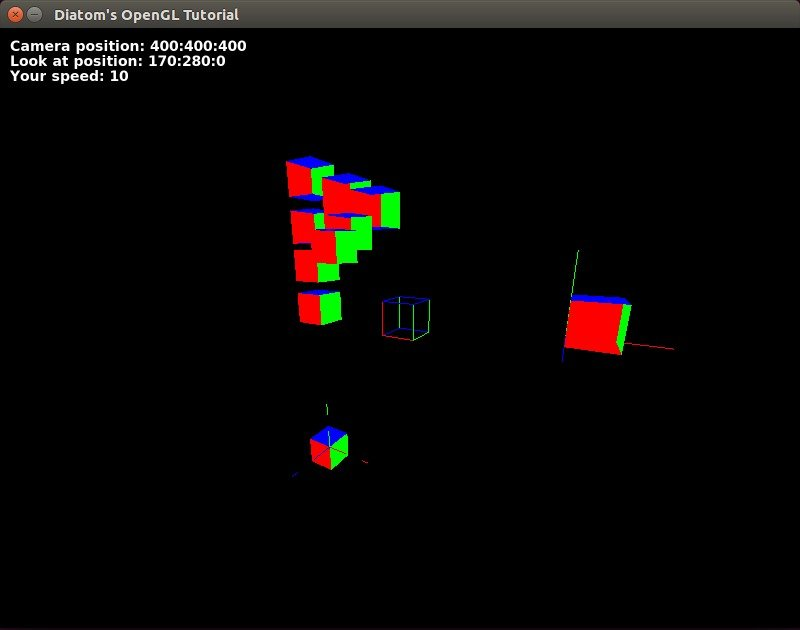Let’s add some textures. We can create a gosu image object from file and then get texture information from it with the `gl_tex_info` method. To build it, let’s add a class ‘texture.’

```class Texture
attr_accessor :info
def initialize(window, filepath)
@image = Gosu::Image.new(window, filepath, {tileable: true, retro: false})
@info = @image.gl_tex_info
# gl_tex_info can return nil if the image was too large to fit onto
# a single OpenGL texture and was internally split up.
end
end

```

Then we will need to update our cube draw method with:

```texture_info = texture.info
glEnable(GL_TEXTURE_2D) # enables two-dimensional texturing to perform
glBindTexture(GL_TEXTURE_2D, texture_info.tex_name) # bing named texture to a target
glPixelStorei(GL_UNPACK_ALIGNMENT,1)
draw_textured_cube
glDisable(GL_TEXTURE_2D)

```

where specify the texture’s edge that will be assigned to that point. Basically, the texture’s left bottom coordinates are (0, 0) and top right coordinates are (1, 1), and we stretch it between the edges of our cube. Code sample for one side:

```glTexCoord2d(0,0); glVertex3d(position.x, position.y, position.z)
glTexCoord2d(1,0); glVertex3d(x_width + position.x, position.y, position.z)
glTexCoord2d(1,1); glVertex3d(x_width + position.x, y_width + position.y, position.z)
glTexCoord2d(0,1); glVertex3d(position.x, y_width + position.y, position.z)

```

```  glEnable(GL_LIGHTING)
glLightfv(GL_LIGHT0, GL_AMBIENT, [0.5, 0.5, 0.5, 1])
glLightfv(GL_LIGHT0, GL_DIFFUSE, [1, 1, 1, 1])
glLightfv(GL_LIGHT0, GL_POSITION, [1, 1, 1,1])
glLightfv(GL_LIGHT1, GL_AMBIENT, [0.5, 0.5, 0.5, 1])
glLightfv(GL_LIGHT1, GL_DIFFUSE, [1, 1, 1, 1])
glLightfv(GL_LIGHT1, GL_POSITION, [100, 100, 100,1])
glEnable(GL_LIGHT0)

```

depth test to depth buffer comparisons

```glEnable(GL_DEPTH_TEST);
glDepthFunc(GL_LEQUAL);

```

and textures with

```glEnable(GL_TEXTURE_2D) # enables two-dimensional texturing to perform
glBindTexture(GL_TEXTURE_2D, texture_info.tex_name) # bing named texture to a target
glPixelStorei(GL_UNPACK_ALIGNMENT,1)

```

we can build nice textured scene with real lighting.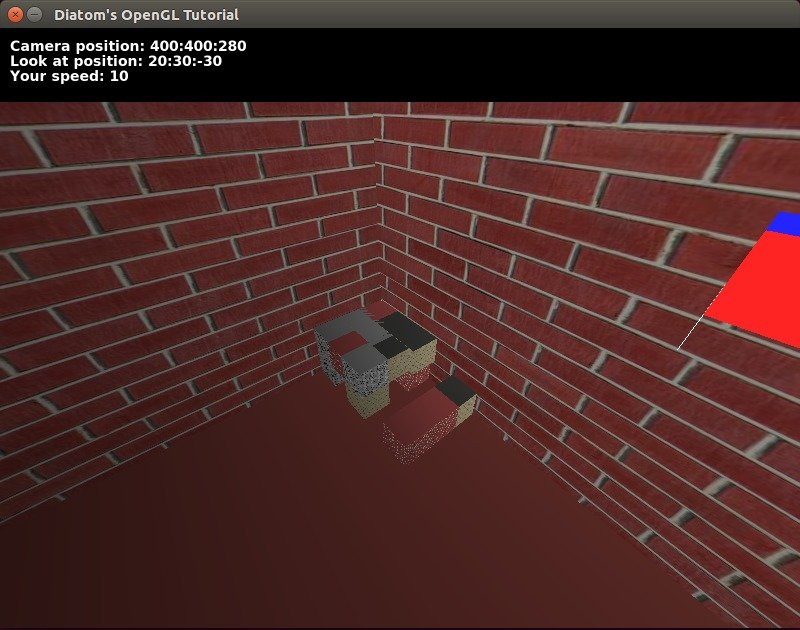With some additional work, we can achieve a switch of material for blocks that we are trying to build and other nice things.